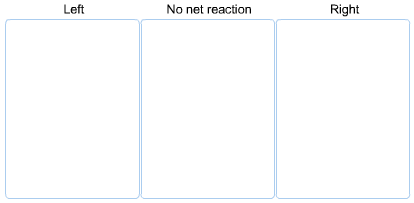# Problem: At 850 K the equilibrium constant for the following reaction is Kc = 15.2SO2(g) + O2(g) ⇌ 2SO3(g)If we mix the following concentrations of the three gases, predict in which direction the net reaction will proceed toward equilibrium.[SO2] = 0.20 M[O2] = 0.60 M[SO3] = 0.60 M[SO2] = 0.14 M[O2] = 0.10 M[SO3] = 0.40 M[SO2] = 0.90 M[O2] = 0.50 M[SO3] = 0.10 M

🤓 Based on our data, we think this question is relevant for Professor Kellman's class at UO.

###### FREE Expert Solution###### Problem Details

At 850 K the equilibrium constant for the following reaction is Kc = 15.

2SO2(g) + O2(g) ⇌ 2SO3(g)

If we mix the following concentrations of the three gases, predict in which direction the net reaction will proceed toward equilibrium.

[SO2] = 0.20 M
[O2] = 0.60 M
[SO3] = 0.60 M

[SO2] = 0.14 M
[O2] = 0.10 M
[SO3] = 0.40 M

[SO2] = 0.90 M
[O2] = 0.50 M
[SO3] = 0.10 MWhat scientific concept do you need to know in order to solve this problem?

Our tutors have indicated that to solve this problem you will need to apply the The Reaction Quotient concept. You can view video lessons to learn The Reaction Quotient. Or if you need more The Reaction Quotient practice, you can also practice The Reaction Quotient practice problems.

What is the difficulty of this problem?

Our tutors rated the difficulty ofAt 850 K the equilibrium constant for the following reaction...as medium difficulty.

How long does this problem take to solve?

Our expert Chemistry tutor, Dasha took 5 minutes and 46 seconds to solve this problem. You can follow their steps in the video explanation above.

What professor is this problem relevant for?

Based on our data, we think this problem is relevant for Professor Kellman's class at UO.